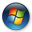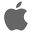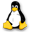## Tuesday, August 5, 2014

### Exporting UF Tables and new expiry

A new version of EulumdatTools 0.11.1.v20140806 was published on August 6 at http://www.fold1.com/eulumdattools/ to replace the non-working 0.11.0.v20140804. In addition to the existing export of "ERCO-like" E/100lux/m2 tables EulumdatTools can now also export "UF" or Utilization Factor tables in line with LiTG publication 3.5 directly from correct EULUMDAT files.
• Export of UF tables with user selectable reflectance triplets for Ceiling, Wall and Working Plane.
• Changed the hard coded expiry date for this version to 2015-01-01.
• The version number was updated to 0.11.0.
The Utilisation Factor method uses the following formula to calculate the illuminance EN: $$E_N = \frac{ LLF . n . \phi . UF }{a . b}$$ The required number of luminaires n can be calculated with the following formula: $$n = \frac{ E_N . a. b }{\phi . UF . LLF}$$ Where
EN
Illuminance in lux
LLF
Light Loss Factor
a
width of space
b
length of space
$$\phi$$
Luminous flux of luminaire
UF
utilization factor from table
n
number of luminaires

•Windows 32 bit OR Windows 64 bit
•Mac OSX Cocoa 32 bit OR Mac OSX Cocoa 64 bit
•Linux GTK 32 bit OR Linux GTK 64 bit